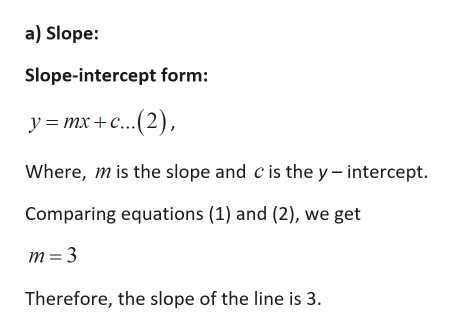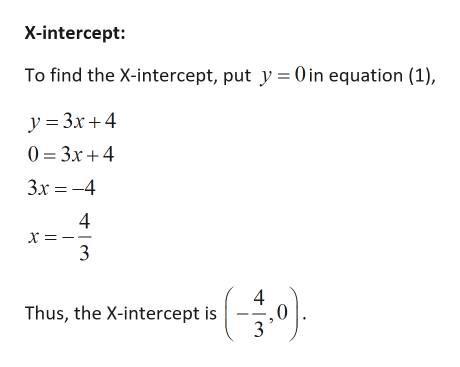# Please help with the following problem:For the liney equals 3 x plus 4  (y=3x+4​)​(a) find the​ slope, and ​(b) sketch the graph.Thank you in advance.

Question
14 views

For the line

y equals 3 x plus 4  (y=3x+4​)

​(a) find the​ slope, and ​(b) sketch the graph.

check_circle

Step 1

Given:

Step 2

Calculation:help_outlineImage Transcriptionclosea) Slope: Slope-intercept form: У 3 тх + с...(2), Where, mis the slope and c is the y - intercept. Comparing equations (1) and (2), we get m 3 Therefore, the slope of the line is 3 fullscreen
Step 3

b) Graph:

To sketch the graph, we have to f...help_outlineImage Transcriptionclosex-intercept: To find the X-intercept, put y 0 in equation (1), y 3x4 0 3x4 3x -4 х - 3 (음아 4 Thus, the X-intercept is 3 fullscreen

### Want to see the full answer?

See Solution

#### Want to see this answer and more?

Solutions are written by subject experts who are available 24/7. Questions are typically answered within 1 hour.*

See Solution
*Response times may vary by subject and question.
Tagged in

### Other